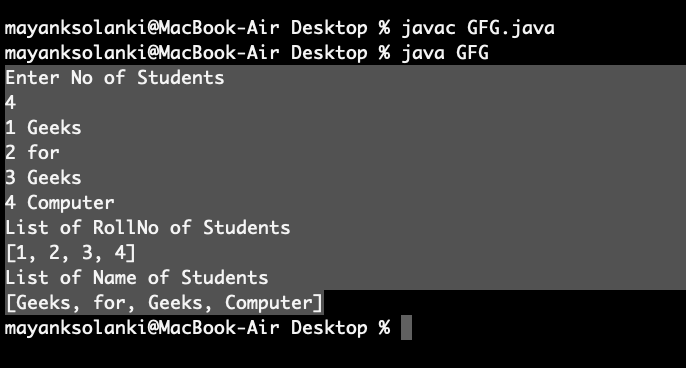# Conversion of Java Maps to List

• Last Updated : 28 Oct, 2021

A Map is an object that maps keys to values or is a collection of attribute-value pairs. The list is an ordered collection of objects and the List can contain duplicate values. The Map has two values (a key and value), while a List only has one value (an element). So we can generate two lists as listed:

• List of values and
• List of keys from a Map.

Let us assume “map” is the instance of Map, henceforth as usual we all know it contains set and value pairs. so they are defined as follows:

• map.values() will return a Collection of the map’s values.
• map.keySet() will return a set of the map’s keys.

Methods:

1. Passing sets of keys inside ArrayList constructor parameter
2. Passing collection of map values generated by map.values() method to the ArrayList constructor parameter
3. Using Streams API (only applicable after JDK 8 and onwards)

Let us discuss each of them

Methods 1: Passing sets of keys inside ArrayList constructor parameter

Procedure: We can convert Map keys to List of Keys bypassing set of map keys generated by map.keySet() method to the ArrayList constructor parameter as shown below

```map.values(); // Now here e will pass sets of keys of our map, so it becomes
map.values(map.keySet());  // Now we just need to store it into List, so creating object
List ListofKeys = new ArrayList(map.keySet()); // Now we are done with conversion. ```

Syntax: Henceforth it as follows:

`List ListofKeys = new ArrayList(map.keySet());`

Method 2: List ListofKeys = new ArrayList(map.keySet());

We can convert Map keys to a List of Values by passing a collection of map values generated by map.values() method to ArrayList constructor parameter.

Syntax: Henceforth it is as follows:

`List Listofvalues = new ArrayList(map.values());`

Example

## Java

 `// Java Program to Convert Map to List` `// Importing required classes``import` `java.util.*;` `class` `GFG {` `    ``// Method 1``    ``public` `static` `void` `main(String[] args)``    ``{` `        ``// Creating HashMap``        ``HashMap hs = ``new` `HashMap<>();` `        ``// Adding elements to hashMap``        ``hs.put(``"Geeks"``, ``1``);``        ``hs.put(``"for"``, ``2``);``        ``hs.put(``"Geeks"``, ``3``);``        ``hs.put(``"Computer"``, ``4``);``        ``hs.put(``"Science"``, ``5``);``        ``hs.put(``"Portal"``, ``6``);` `        ``// Calling method``        ``MapValuesToList obj = ``new` `MapValuesToList(hs);` `        ``// Storing into List``        ``List mapvaltolist = obj.mapvaltolist(hs);` `        ``// Printing via for loops``        ``for` `(Integer integerList : mapvaltolist) {` `            ``// Printing our ArrayList``            ``System.out.println(integerList);``        ``}``    ``}` `    ``// Method 2``    ``// To convert Map to List``    ``public` `List``    ``mapvaltolist(Map map)``    ``{` `        ``// Using Collection``        ``Collection val = map.values();` `        ``// Creating an ArrayList``        ``ArrayList al = ``new` `ArrayList<>(values);` `        ``return` `al;``    ``}``}`

Output:

```1
2
3
4
5
6```

Method 3: Using Streams API

The stream() method returns a stream of the keys from the Set of the map keys returned by Map.keySet(). The collect() method of the Stream class collects the stream of keys in a List.The Collectors.toCollection(ArrayList::new) passed to the collect() method to collect as new ArrayList. One can also use Stream API to convert map keys and values to respective lists. the syntax is as provided below as follows:

`List ListofKeys = map.keyset().stream().collect(Collectors.toCollection(ArrayList::new));`
`List Listofvalues= map.values().stream().collect(Collectors.toCollection(ArrayList::new));`

Note: You can collect elements of Stream in an ArrayList, LinkedList, or any other List implementation.

Implementation:

Given RollNo and Student Name of N students as input. First, we create a Map where Rollno is key because Rollno is unique and Name as Value for Map then convert this map to list of values and keys respectively. Where the generated list of keys contains RollNo of students and list of Values contains Name of Students.

Example

## Java

 `// Java program to Convert Map to List` `// Importing required classes``import` `java.util.*;``// Importing stream sub-package``import` `java.util.stream.*;` `// Main class``// MapToList``class` `GFG {` `    ``// Main driver method``    ``public` `static` `void` `main(String[] args)``    ``{` `        ``// Scanner class to take input of key-value pairs``        ``Scanner sc = ``new` `Scanner(System.in);` `        ``// Creating a Hashmap which maps rollno with student``        ``// name``        ``Map map``            ``= ``new` `HashMap();` `        ``// Command for better usability``        ``System.out.println(``"Enter No of Students"``);` `        ``// Taking input to Hashmap``        ``// via iterating using for loop``        ``int` `noOfStudents = Integer.parseInt(sc.nextLine());``        ``for` `(``int` `i = ``0``; i < noOfStudents; i++) {` `            ``String input = sc.nextLine();``            ``String[] studentdata = input.split(``" "``);` `            ``String rollno = studentdata[``0``];``            ``String name = studentdata[``1``];` `            ``// Simply inserting received pairs to Map``            ``map.put(rollno, name);``        ``}` `        ``// Now first create list of keys and values``        ``List ListofKeys = ``null``;``        ``List ListofValues = ``null``;` `        ``// Now converting hashMap to List of keys and values``        ``ListofKeys = map.keySet().stream().collect(``            ``Collectors.toCollection(ArrayList::``new``));``        ``ListofValues = map.values().stream().collect(``            ``Collectors.toCollection(ArrayList::``new``));` `        ``// lastly printing List of rollno and name of``        ``// students``        ``System.out.println(``"List of RollNo of Students"``);``        ``System.out.println(ListofKeys.toString());` `        ``System.out.println(``"List of Name of Students"``);``        ``System.out.println(ListofValues.toString());``    ``}``}`

Output:My Personal Notes arrow_drop_up